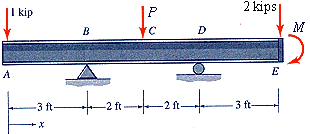# Beam equilibrium and shear force

bcd201115

## Homework Statement

transverse loads are applied to the beam in the figure at A, C, and E, and a concentrated couple 3Pa is applied to the beam at E. Determine expressions for (a) the transverse shear force Vc and bending moment Mc at a section just to the left of the load at C, and (b) shear Vd and moment Md just to the left of the support at D. Express answers in terms of P and a.

The image is the same setup as this problem only the loads at A, C and E are P, 2P and 2P respectively## Homework Equations

I know that the derived equations are V=dM/dx and p(x)=dV/dx but I don't really know how to go about doing this. I have my free body diagram but other than that I don't know where to start. Can anyone give me a hand?

Staff Emeritus